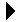Integer Stack with Exceptions# Integer Stack with ExceptionsData Exception with Continuation Example Download Integer Stack with Exceptions Body```--
-- Basic array-based stack modified to send exceptions on overflow and
-- underflow.
--
-- A simple array-based stack package.  Of course, it has a size
-- limit.  The operations are what you would expect:
--   Int_Stack  : Type of stack variables.
--   Push       : Push the integer on the stack.
--   Pop        : Pop the integer and place the result in the parameter.
--                If empty, puts zero and leaves stack unchanged.
--   Top        : Return the top of the stack, or 0 if there is none.
--   Empty      : See if the stack is empty.
--   Full       : See if the stack is full.
--   Clean      : Make the stack empty.
--
package I_Stack2 is
-- This declares that Int_Stack is a type, but the contents of the type
-- are private, and are invisible to clients of the package.
type Int_Stack is private;

-- Here are the exceptions.
Stack_Underflow, Stack_Overflow: exception;

-- Max stack size.
Max_Size: constant Integer := 10;

-- All the public stack operations.
procedure Push(S: in out Int_Stack; I: Integer);
procedure Pop(S: in out Int_Stack; I: out Integer);
function Top(S: Int_Stack) return Integer;
function Empty(S: Int_Stack) return Boolean;
function Full(S: Int_Stack) return Boolean;
procedure Clean(S: in out Int_Stack);

private
-- The items here cannot be accessed by clients of the package.
type Stack_Data_Type is array(1..Max_Size) of Integer;
type Int_Stack is record
Size: Integer := 0;
Data: Stack_Data_Type;
end record;
end I_Stack2;
```Data Exception with Continuation Example Integer Stack with Exceptions Body